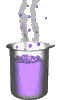Science class online
Chemistry and physics lessons

# Mechanics

Free online mechanics lesson for elementary school,  middle school and high school.Science class Chemistry Electricity Optics Mechanics Mechanics lessons Gravity - The solar system - motions of celestial bodies - Physical interactions - Effects of gravity - Weight of an object - Difference between weight and mass - Relationship between weight and mass  Energy - Energy basics - Potential energy - Kinetic energy - Mechanical energy - Translational Kinetic Energy - kinetic energy mass and speed

 _____________________________________________________   _____________________________________________________

## Gravity

### Translational kinetic energy

 1) What is translational motion ? If an object is as small as a point ( it has only one dimension ), it always has translational motion As for other objects, they have a translational motion if each of their points: - move at the same speed. - move in the same direction. For simplification we can say that an object has a translational motion if it doesn't rotate. 2) kinetic energy formula Translational kinetic energy ( KE ) depends on speed ( v ) and mass ( m ) of the moving object. It can be expressed by the following relationship: KE = 1 x m x v2           2 - m  in kilogram (kg) - v in meter per second (m/s) - Ec in joule (J) ______________________________________ ______________________________________
____________________________________

____________________________________